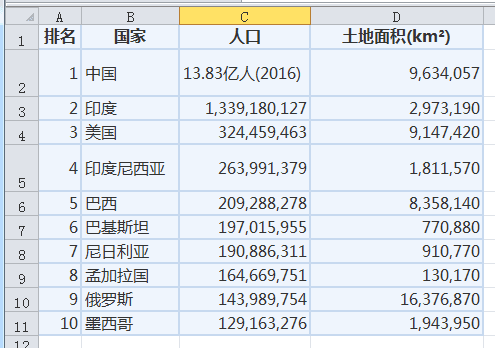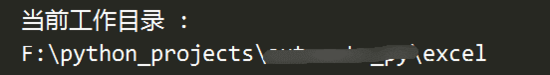# Python处理Excel文件（openpyxl模块）--特别好用！！！

Excel表格在我们日常办公中是不可或缺的，学会用python处理excel表格对我们工作效率会有很大提升。python提供的xlrd模块其实并不好用，它会有容量限制，我发现了一个比较好用的模块——openpyxl，它的容量大，而且简单易用。接下来我就讲一下它的一些常用的基本操作。

### 1、Excel 基础概念

Excel 文件也称做为工作簿。每个工作簿可以包含多个工作表(Sheet)。用户当前查看的表或关闭 Excel 前最后查看的表，称为活动表。

### 2、安装 openpyxl

pip install openpyxl


### 3、读取 Excel3.1 加载

wb = openpyxl.load_workbook('population.xlsx')
print('wb 类型 :')
print(type(wb))


wb 类型 :
<class 'openpyxl.workbook.workbook.Workbook'>


import os
print('当前工作目录 :')
print(os.getcwd())3.2 读取 Excel 工作表（sheet）

• Workbook 对象的 sheetnames 属性可以获取所有 sheet 表的表名列表。
• Workbook 对象的 active 属性，会获取当前活动表，即打开 Excel 时出现的工作表。
• 获取 Worksheet 对象后，我们就可以通过 title 属性得到 sheet 的名称。
...
print('取得所有工作表的表名 :')
print(wb.sheetnames)

print('取得某张工作表 :')
sheet = wb['Sheet3']
print(type(sheet))
print('表名 - ' + sheet.title)

print('取得活动工作表 :')
active_sheet = wb.active
print('表名 - ' + active_sheet.title)


取得所有工作表的表名 :
['Sheet1', 'Sheet2', 'Sheet3']

<class 'openpyxl.worksheet.worksheet.Worksheet'>
表名 - Sheet3



3.3 读取单元格（Cell）

• Cell 对象的 value 属性，存放的是该单元格中所保存的值。
• Cell 对象的 row、 column 和 coordinate 属性，存放的是该单元格的位置信息。
• 用字母来指定列比较奇怪，因此我们也可以通过 sheet 的 cell 方法直接指定行（row）与列（column），来获取单元格 Cell 对象。
...
print('取得 A1 单元格 :')
cell = active_sheet['A1']
print(cell)
print(cell.value)

print('取得 B1 单元格 :')
cell = active_sheet['B1']
print(cell)
print(cell.value)

print('行号为 ' + str(cell.row) + ',列号为 ' + str(cell.column) + ' 的单元格，其值为 ' + cell.value)
print('单元格 ' + cell.coordinate + ' 其值为 ' + cell.value)

print('取得 C1 单元格的值 :')
print(active_sheet['C1'].value)

print('通过指定行与列，来获取单元格:')
print(active_sheet.cell(row=1, column=2))
print(active_sheet.cell(row=1, column=2).value)

print('迭代行与列，来获取单元格的值:')
for i in range(1, 8, 2):
print(i, active_sheet.cell(row=i, column=2).value)


取得 A1 单元格 :
<Cell 'Sheet1'.A1>

<Cell 'Sheet1'.B1>

<Cell 'Sheet1'.B1>

1 国家
3 印度
5 印度尼西亚
7 巴基斯坦


3.4 获取工作表大小
Worksheet 对象的 max_row 与 max_column，可以获取工作表的总行数与总列数，即工作表的大小。

print('获取工作表的大小:')
print('总行数 -> ' + str(active_sheet.max_row))
print('总列数 -> ' + str(active_sheet.max_column))


获取工作表的大小: 总行数 -> 11 总列数 -> 4


3.5 列转换函数
openpyxl 提供了两个函数，用于转换列号：

• openpyxl.utils.get_column_letter -> 会把数字转化为字母。
• openpyxl.utils.column_index_from_string -> 会把字母转化为数字。
import openpyxl
from openpyxl.utils import get_column_letter, column_index_from_string
...
print('列转换函数:')
print('[数字转换为字母]')
print('第 1 列 -> ' + get_column_letter(1))
print('第 2 列 -> ' + get_column_letter(2))
print('第 37 列 -> ' + get_column_letter(37))
print('第 818 列 -> ' + get_column_letter(818))
print('[字母转换为数字]')
print('第 A 列 -> ' + str(column_index_from_string('A')))
print('第 CC 列 -> ' + str(column_index_from_string('CC')))


列转换函数:
[数字转换为字母]

[字母转换为数字]



3.6 切片

print(tuple(active_sheet['A2':'D4']))
for row_objects in active_sheet['A2':'D4']:
for cell_object in row_objects:
print(cell_object.coordinate, cell_object.value)
print('-- 当前行获取结束 --')


((<Cell 'Sheet1'.A2>, <Cell 'Sheet1'.B2>, <Cell 'Sheet1'.C2>, <Cell 'Sheet1'.D2>), (<Cell 'Sheet1'.A3>, <Cell 'Sheet1'.B3>, <Cell 'Sheet1'.C3>, <Cell 'Sheet1'.D3>), (<Cell 'Sheet1'.A4>, <Cell 'Sheet1'.B4>, <Cell 'Sheet1'.C4>, <Cell 'Sheet1'.D4>))
A2 1
B2 中国
C2 13.83亿人(2016)
D2 9634057
-- 当前行获取结束 --
A3 2
B3 印度
C3 1339180127
D3 2973190
-- 当前行获取结束 --
A4 3
B4 美国
C4 324459463
D4 9147420
-- 当前行获取结束 --

• 这里首先通过 tuple() 方法，展示出了切片后的所有 Cell 对象。
• 然后使用了两个 for 循环，外层 for 循环会遍历这个切片中的每一行；而内层 for 循环会遍历该行中的每个单元格。

3.7 获取指定行或指定列

print('获取特定行:')
print(list(active_sheet.rows))
for cell_object in list(active_sheet.rows):
print(cell_object.value)

print('获取特定列:')
print(list(active_sheet.columns))
for cell_object in list(active_sheet.columns):
print(cell_object.value)


获取特定行:
(<Cell 'Sheet1'.A3>, <Cell 'Sheet1'.B3>, <Cell 'Sheet1'.C3>, <Cell 'Sheet1'.D3>)
2

1339180127
2973190

(<Cell 'Sheet1'.C1>, <Cell 'Sheet1'.C2>, <Cell 'Sheet1'.C3>, <Cell 'Sheet1'.C4>, <Cell 'Sheet1'.C5>, <Cell 'Sheet1'.C6>, <Cell 'Sheet1'.C7>, <Cell 'Sheet1'.C8>, <Cell 'Sheet1'.C9>, <Cell 'Sheet1'.C10>, <Cell 'Sheet1'.C11>)

13.83亿人(2016)
1339180127
324459463
263991379
209288278
197015955
190886311
164669751
143989754
129163276

• Worksheet 对象的 rows 或者 columns 属性，都会返回一个由 “行元组” 构成的元组。每个行元组都拥有该行中的所有Cell 对象。
• 可以通过行元组的下标，来访问具体的 Cell 对象。

### 4、写入 Excel

data = open('./data.txt', 'r')
outwb = openpyxl.Workbook()  # 打开一个将写的文件
outws = outwb.create_sheet(index=0)  # 在将写的文件创建sheet

i = 1  # 注意：'cell'函数中行列起始值为1
for line in data:
for x in range(0,len(line)):
outws.cell(column = x+1 , row = i , value = "%s" % line[x])
i += 1
savexlsx = "./results.xlsx"
outwb.save(savexlsx)  # 保存结果
data.close()10-30
07-03
07-31633
07-10822
06-051732
02-142340
04-164403